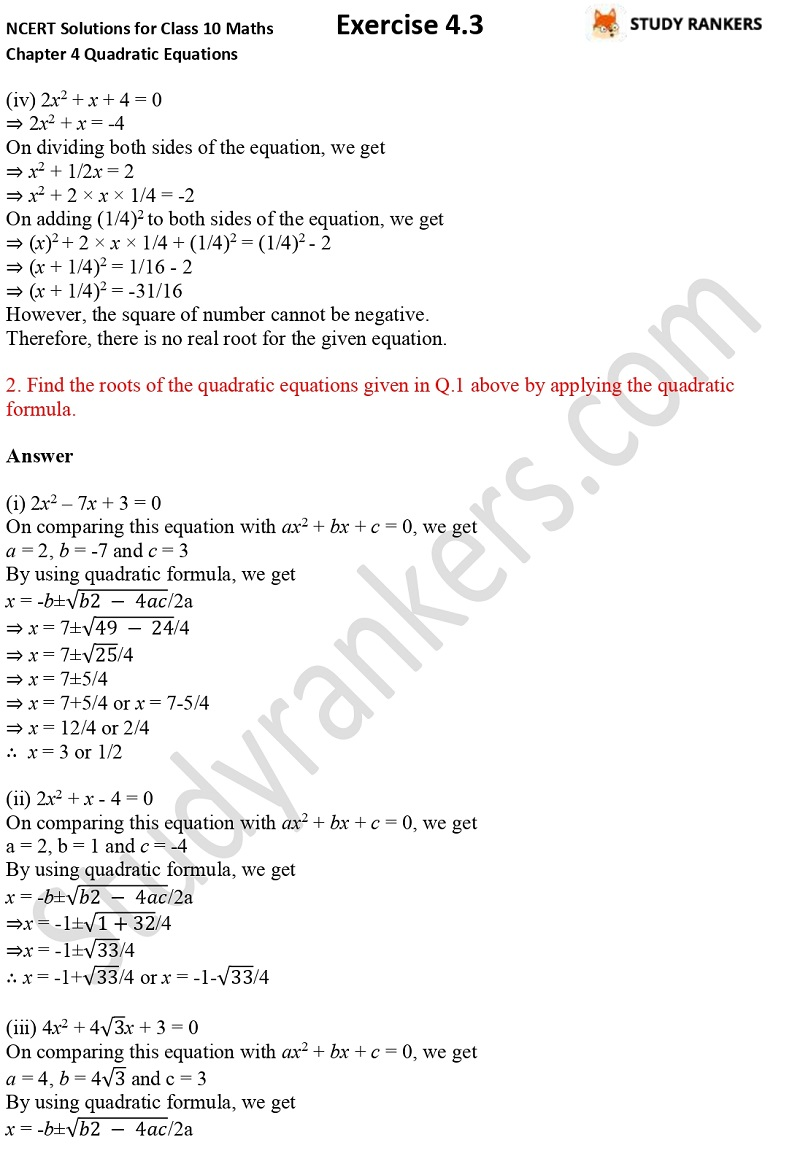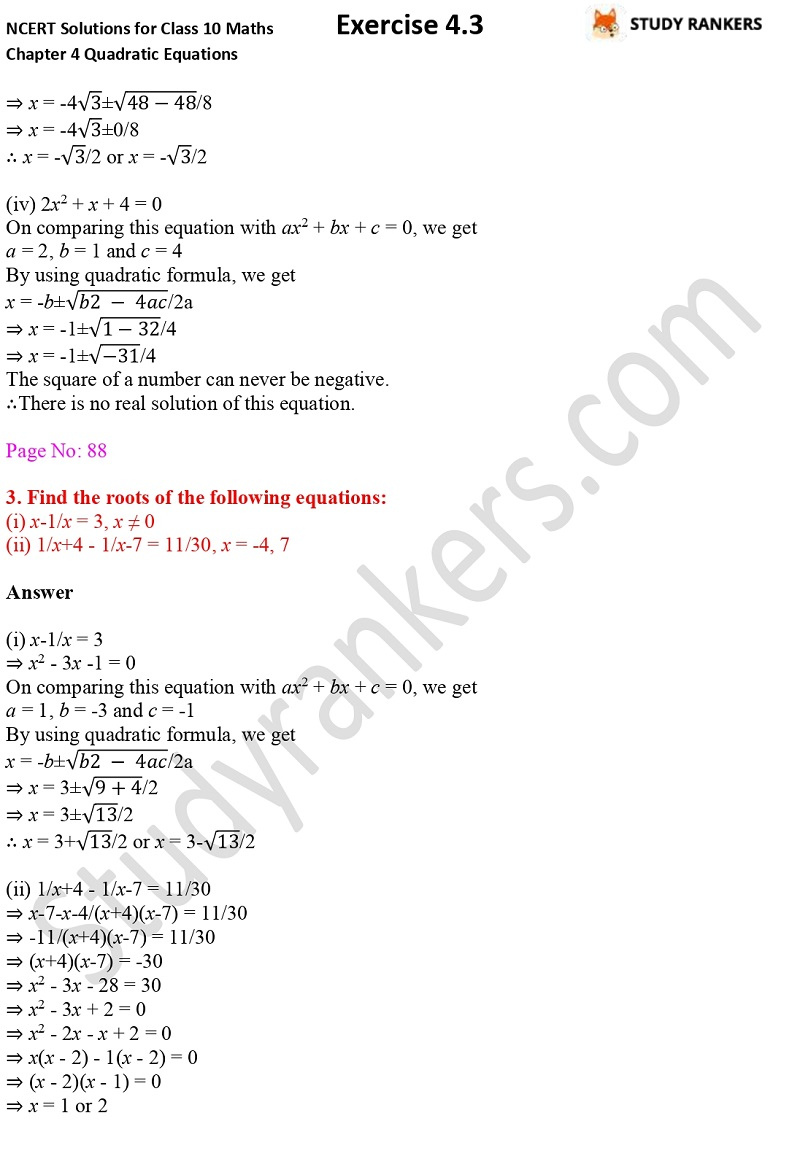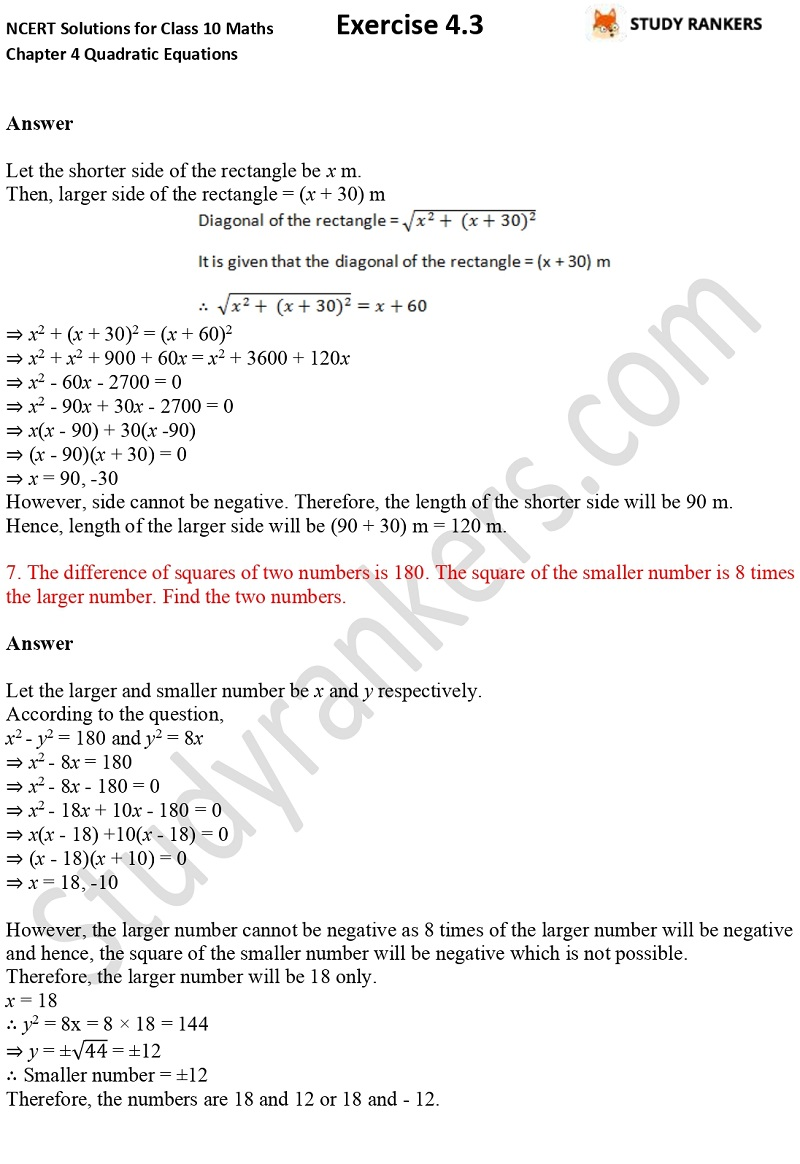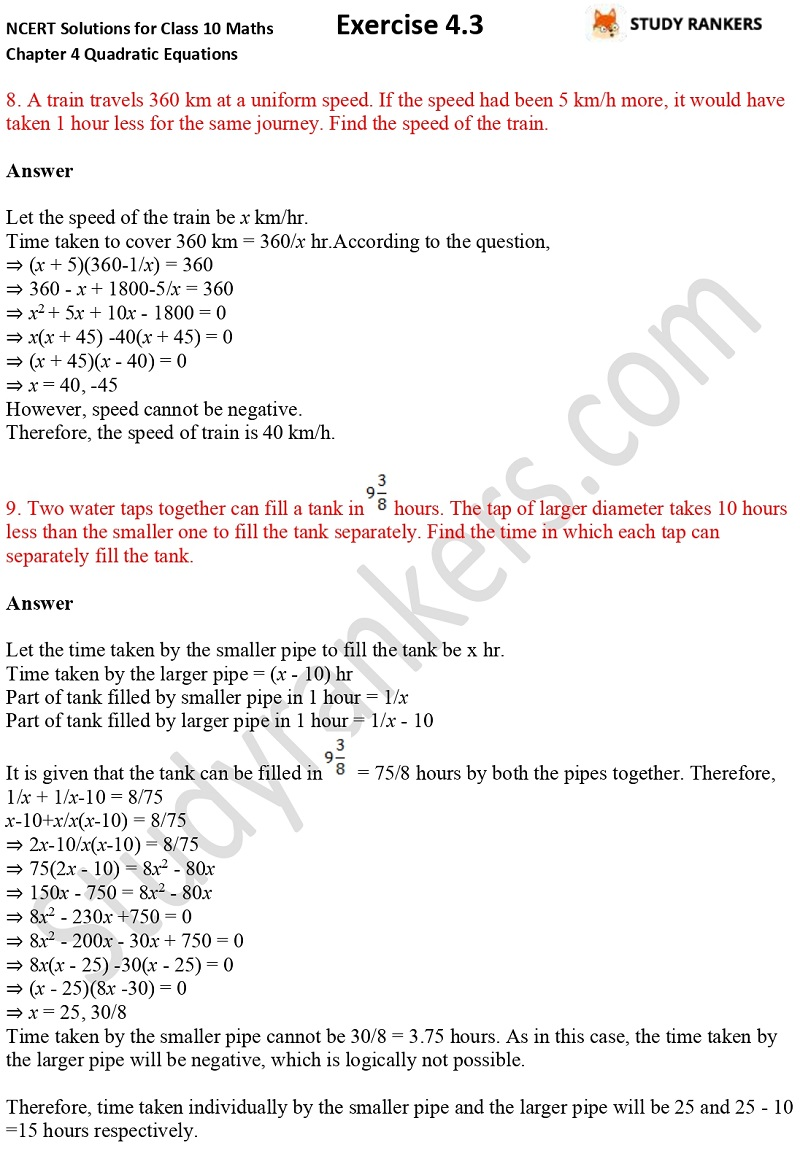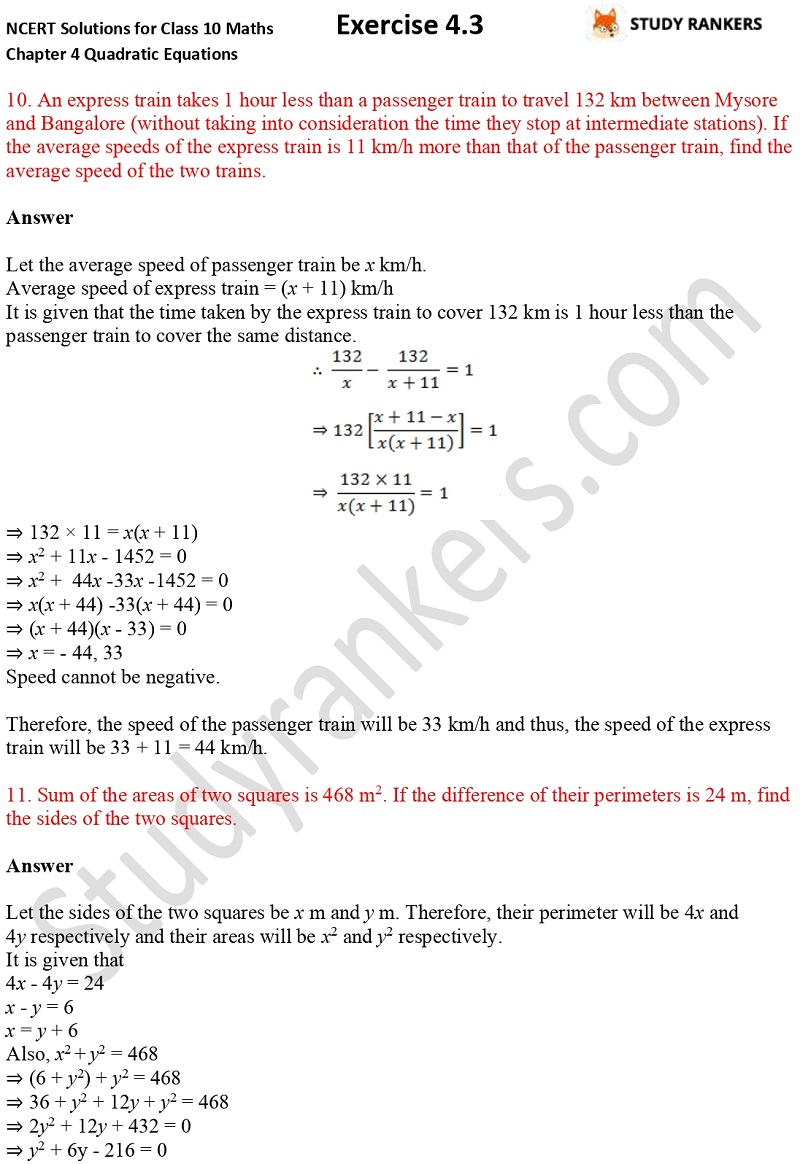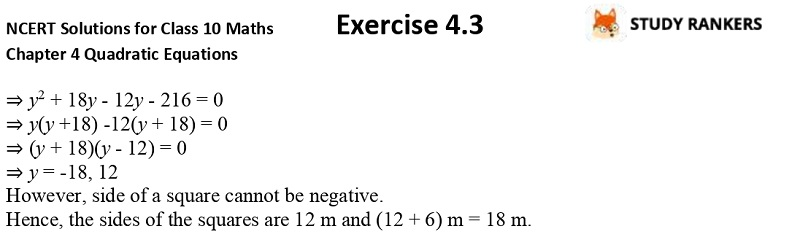>

## NCERT Solutions for Class 10 Maths Chapter 4 Quadratic Equations Exercise 4.3

Here you can find Chapter 4 Quadratic Solutions NCERT Solutions for Class 10 Maths Exercise 4.3 which will provide you with the concepts of solving questions from basic to advance. You need to solve these questions first before going for advance level Maths books. These CBSE NCERT solutions are updated as per the latest NCERT Textbooks. Math Class 10 Solutions will be helpful in developing problem solving skills and boosting student's confidence.

Exercise 4.3 has total eleven questions which are very important for the purpose of exams. You have to find the roots of the given quadratic equations by the method of completing the square. There are variety of word problems also provided.